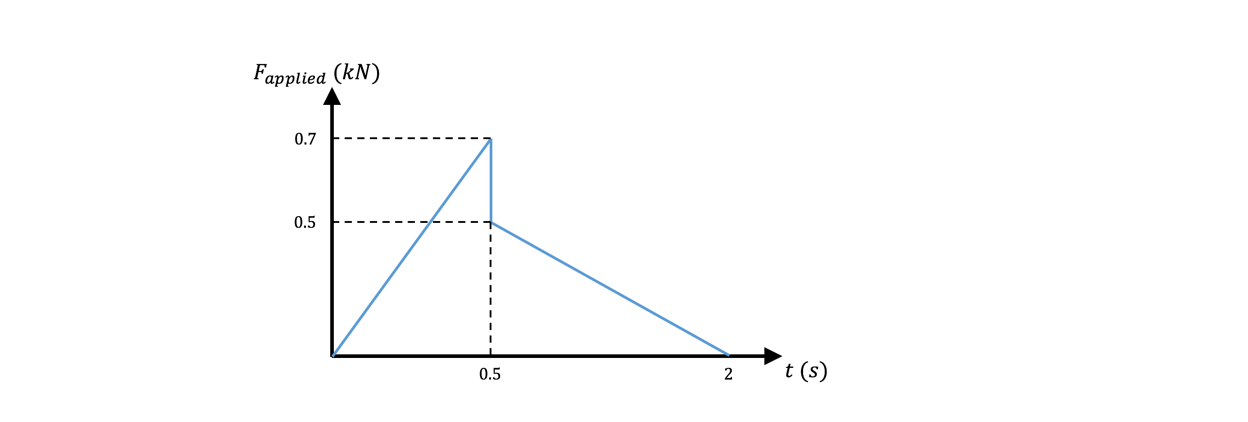# Problem: Consider the figure below, graphing the force applied on a 200 kg box vs time. During the first 0.5 s on the graph, the box remains at rest, while during the remaining time on the graph, the box moves at a constant speed of 15 m/s. At what time would the force of static friction be 0.5 kN?

###### FREE Expert Solution
91% (480 ratings)
###### Problem Details

Consider the figure below, graphing the force applied on a 200 kg box vs time. During the first 0.5 s on the graph, the box remains at rest, while during the remaining time on the graph, the box moves at a constant speed of 15 m/s. At what time would the force of static friction be 0.5 kN?Frequently Asked Questions

What scientific concept do you need to know in order to solve this problem?

Our tutors have indicated that to solve this problem you will need to apply the Static Friction concept. You can view video lessons to learn Static Friction. Or if you need more Static Friction practice, you can also practice Static Friction practice problems.

How long does this problem take to solve?

Our expert Physics tutor, Patrick took 5 minutes and 4 seconds to solve this problem. You can follow their steps in the video explanation above.